### TERRESTRIAL ATMOSPHERE MODELLING

INTRODUCTION

Atmospheric density is a critical component for studies of meteor flight, artificial space debris reentry and space object (satellite and debris) lifetime.

Atmospheric density varies with altitude, geographical latitude and longitude, time, season and atmospheric dynamics. In the upper atmosphere (>100 km) it also depends strongly on the solar soft x-ray and extreme ultraviolet (EUV) output. It also varies according to the geomagnetic activity. In the lower atmosphere a mean density as a function of altitude is normally sufficient for models of meteors and space debris reentry. In the upper atmosphere for lifetime studies which consider atmospheric drag the density must be specified as a function of altitude, solar ten centimetre flux and the geomagnetic activity index Ap.

The following sections will consider a number of simple atmospheric density models that are useful for the above applications.

AN ISOTHERMAL ATMOSPHERE

An isothermal atmosphere is one that has a constant temperature. In such an atmosphere the density (ρ) can be written as

ρ = ρo exp ( - h / H )
where
ρo is the density at the surface of the planet
h is the height above the surface
H is the scale height
The scale height H is the height at which the density falls to a value of 1/e of the surface density. It is furthermore the height at which the entire atmosphere would fit if it had a constant density ρo up to this height. H is also a function of the atmospheric temperature T and the mean molecular mass m:
H = ( k T ) / ( m g )
where
k = 1.38 x 10-23 J/K is Boltzmann's constant
g is the acceleration due to gravity (~9.8 at Earth's surface)
Although the temperature of the Earth's atmosphere varies does vary with height, it is still possible to use the isothermal approximation over limited height ranges. Below 100 km the composition of the atmosphere is relatively constant (20% molecular oxygen and 80% molecular hydrogen) so the mean molecular mass is constant. The region of the atmosphere is referred to as the homogenosphere. Above 100km the constituents of the atmosphere change and this is referred to as the heterogenosphere.

Below 100km the variation of gravity with altitude is insignificant. Thus the only factor that varies significantly is the temperature.

THE REAL ATMOSPHERE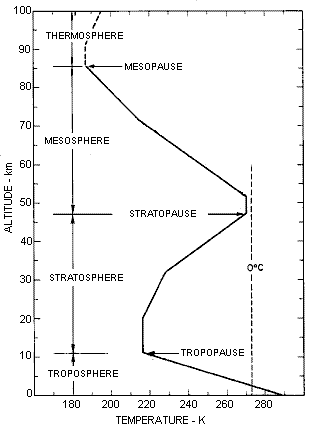The graph at the left shows the temperature variation with height that is used in the US Standard Atmosphere model. It is a mean of mostly mid-latitude conditions in the northern hemisphere. Some is derived from extensive data (eg below 20 km). Some is derived from quite limited data (eg between 50 and 80 km). From the surface the temperature intially decreases at what is called the standard adiabatic lapse rate of about 7 degrees per kilometre. It then plateaus at -55 Celcius for almost 10 km, before rising again to another plateau just below 0 C. It decreases again in the Mesosphere before finally rising when it reaches the Thermosphere. The changing temperature causes the scale height to change. From the surface to 100 km altitude H varies between about 5.5 and 8.0 km , rising and falling along with the temperature.

Despite the changing scale height, a simple isothermal model with a fixed scale height can represent the atmosphere up to 100 km with an error less than 50%, and up to 130 km with an error no larger than a factor of 2. These errors are quite comparable to the variation that the real atmosphere shows from day to day from the mean 'standard' model. This model is quite suitable for demonstrating the physics of meteor flight and space debris reentries. High velocity meteoroids (~60 km/s) start ablating around 130 km altitude, but space debris reentries, with the much lower velocity of ~ 8 km/s only start to show significant atmospheric interaction below 90 km.

The simple isothermal model is specified by the formula:

ρ = 1.3 exp ( -h / 7000 )     kg m-3
where h is the altitude in metres. The comparison of the density from this model with the CIRA model (see next section) is given in the table at right.
 ``` Height Isothermal Standard 0 1.30E+00 1.17E+00 10 3.12E-01 4.62E-01 20 7.47E-02 8.67E-02 30 1.79E-02 1.66E-02 40 4.29E-03 4.05E-03 50 1.03E-03 1.17E-03 60 2.46E-04 3.38E-04 70 5.90E-05 8.58E-05 80 1.41E-05 1.79E-05 90 3.39E-06 3.20E-06 100 8.12E-07 5.47E-07 110 1.95E-07 1.03E-07 120 4.67E-08 2.49E-08 130 1.12E-08 8.23E-09 ```

THE CIRA MODEL

The CIRA model is the COSPAR International Reference Atmosphere. COSPAR is the United Nations Committee for Space Research. Graphs of CIRA atmospheric temperature and density to 500 km altitude are shown below: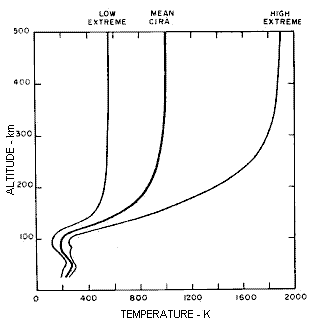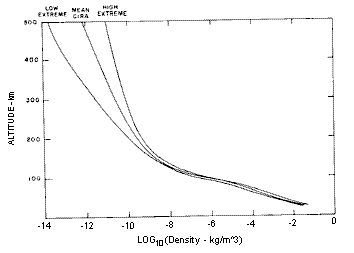The changing temperature is the predominant parameter that changes the scale height and hence the density. However, this is reinforced by the decrease in gravity (g) with increasing altitude and also the reduction in mean molecular mass above 100 km altitude. Below 100km the Sun has only a minor influence on the density. Above 200 km it becomes quite significant. This is because the solar EUV and soft X-rays which affect the upper atmospheric density are absorbed around the 100 km altitude level.

MODELS TO 180 KM

- We can use the mean CIRA model to 180 km by specifying the density values every 5 or 10 km. Because the density varies over many orders of magnitude over this range it is more useful to use the logarithm (base 10) of these values. Linear interpolation can be used to calculate the values intermediate between the specified points at the 10 km intervals. The actual density can then be calculated by raising the interpolated log values as a power of ten. That is, ρ = 10logval.

An alternative is to fit a polynomial to the log points such that:

log10(ρ) = polyfn( h )
which gives:
ρ = 10polyfn( h )
The density (kg m-3) and log(density) values for the mean CIRA model are given in the table at right. Note the difference between this model and the 'standard' model listed in the previous table. A seventh order polynomial fit with the polynomial coefficients is shown in the graph below. The function is given by:
polyfn(h) = a0 + a1*h + a2*h2 + a3*h3 + a4*h4 + a5*h5 + a6*h6 + a7*h7
= ((((((a7*h + a6)*h + a5)*h + a4)*h + a3)*h + a2)*h + a1)*h + a0
[The lower form is identical but computationally more efficient]
 ``` CIRA ATMOSPHERIC DENSITY Height CIRA logCIRA 0 1.21E 0 0.083 10 4.19E-1 -0.38 20 9.39E-2 -1.03 30 1.77E-2 -1.75 40 3.97E-3 -2.40 50 1.06E-3 -2.97 60 3.21E-4 -3.49 70 8.77E-5 -4.05 80 1.91E-5 -4.72 90 3.40E-6 -5.47 100 5.30E-7 -6.28 110 9.66E-8 -7.02 120 2.44E-8 -7.61 130 8.48E-9 -8.07 140 3.85E-9 -8.41 150 2.07E-9 -8.68 160 1.24E-9 -8.91 170 8.04E-10 -9.09 180 5.46E-10 -9.26 ```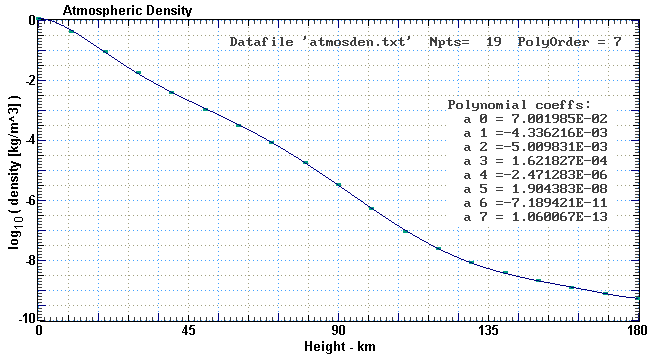A MODEL FROM 180 to 500 KM

Above 180 km we can no longer use a mean reference model. We need a model that varies according to the space weather conditions at the time, specifically the solar EUV flux and the geomagnetic activity. We use the solar radio ten centimetre flux (F10) as a proxy for the solar EUV output, and the geomagnetic Ap index as a proxy for the geomagnetic activity. The model we have developed is suitable for investigating orbital decay and satellite and debris lifetimes in the altitude range from 180 to 500 km.

The algorithm to calculate the atmospheric density in this range is given by:

 T = 900 + 2.5 (F10 ñ 70) + 1.5 Ap (Kelvin) μ = 27 ñ 0.012 (h ñ 200) 180 < h (km) < 500 H = T / μ (km) ρ = 6x10-10 exp( - (h ñ175) / H ) ( kg m-3)
Note that T, μ and H should be regarded as intermediate values in the computation of density. They are 'pseudo' parameters and do not necessarily correspond to temperature, molecular weight and scale height at the specified altitude. Only the final parameter ρ should be regarded as a model output.

Values of the model atmospheric density are graphed below for the cases of minimal (F10=70) and maximal (F10=300) solar EUV and soft X-ray output: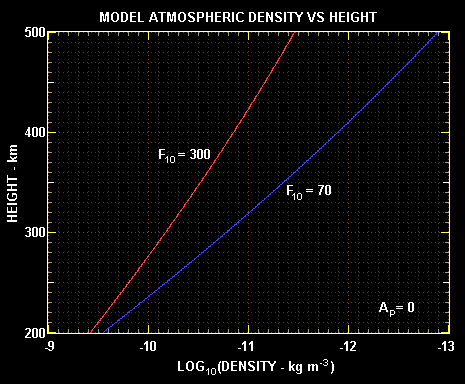Note: it has been found that an average F10 value is the more appropriate parameter to use rather than the daily value. Typically the last 90 days are used to form the average.

Above 500 km altitude the lifetime of an orbital body is larger than the solar period of ~11 years, and so once again an average or mean atmospheric model may be appropriate.

REFERENCE

Adolph S Jursa (Ed.), Handbook of Geophysics and the Space Envvironment, US Airforce Geophysics Laboratory, 1985 {This text can be obtained from the National Technical Information Service, 5285 Port Royal Road, Springfield, VA, 22161 - Document Accession Number: ADA 167000}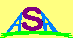Australian Space Academy